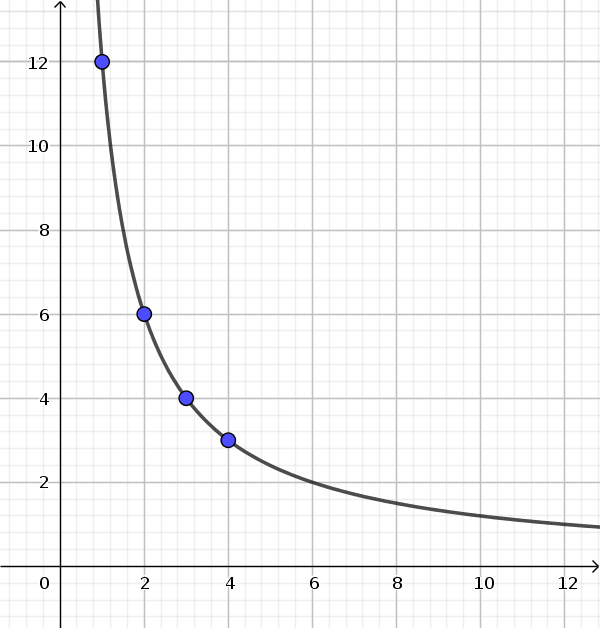Math Relationships Inversely proportional relationship

# Inversely proportional relationship

Inversely proportional relationships are "the more the less"-relationships.

They always decrease evenly (proportional).

### Example

A worker needs 12 days. The number of days required decreases equally with increasing numbers of workers.

Workerneeded days
112
26
34
43

The graph is a hyperbola. It approaches the two axes more and more without reaching them.!

### Remember

If one doubles (triples, quadruples, ...) an initial value, the assigned value halves (thirds, quarters, ...).

## Constant of inverse proportionality (total size)

If we multiply the assigned size times the initial size in an inversely proportional relationship, we always get the same value.

### Example

Workerneeded days
112
26
34
43

$1\cdot12=\color{blue}{12}$
$2\cdot6=\color{blue}{12}$
$3\cdot4=\color{blue}{12}$
$4\cdot3=\color{blue}{12}$

The constant of inverse proportionality is here 12. It is therefore an inversely proportional relationship.

!

### Remember

The constant of inverse proportionality or the total size is the product of initial value (x) and assigned value (y).